## Sunday, June 13, 2010

### Irodov Problem 3.44In this problem first we need to find the electric field due to an infinite thin plane sheet with a hole. For this, we shall first use the superposition principle. The idea being that the electric field due to a sheet with cavity is same as the summation of fields due to a disc with negative charge density and a solid infinite plane sheet with positive charge density as shown in figure 1.First let us determine the electric field due to a charged disc. Consider an infinitesimally thin ring section on the disc (as shown in Figure 2) of thickness dr and radius r.The surface area of this ring will be. The charge contained in this infinitesimally thin ring will thus be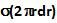. We have already derived the expression for the electric field due to a charged ring at a distance x along the axis from Problem 3.9 Equation 4. Using this expression we have the electric field dE due to this infinitesimally thin ring as(1) thus, gives the electric field due to a positively charged disc at a distance x along the axis of the disc.Now let us determine the elxtric field due to an infinitely large thin charged sheet. For this we can use Gauss law. Consider a Gaussian surface that is in the shape of a cylinder with its axis perpendicular to the sheet and having and area of cross-section A. The total charge contained within this Gaussian cylinder is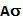. The electric field E will be perpendicular to the Gaussian cylinder at both its ends. Hence we have,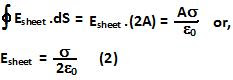The electric field due to the infinite sheet with a circular hole can now be determined as,Now using (3) we can determine the electric field due to two such sheets, one negatively charged and the other positively charged separated by a distance of l from each other (as shown in Figure 4) as,When l is very small we can use Taylor's series to approximate (4) as,When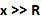(5) becomes,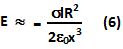Now the electric field potential can be determined as,Whenwe have,